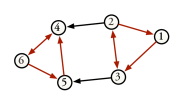# 1.5 Cyclic Graph

A cycle is a simple path that begins and ends at the same vertex and has a length of at least one. We refer to any graph that contains a cycle as a cyclic graph.

Figure 3 illustrates the cycles that are present in the directed graph of Figure 1.

Figure 3: Example of Cycles in a Digraph